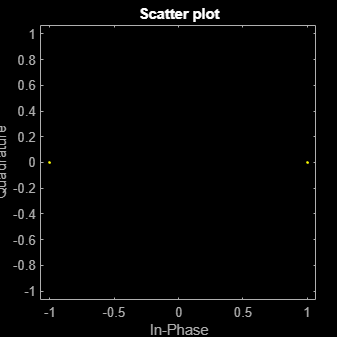# wlanConstellationMap

Constellation mapping

## Syntax

``y = wlanConstellationMap(bits,numBPSCS)``
``y = wlanConstellationMap(bits,numBPSCS,phase)``

## Description

example

````y = wlanConstellationMap(bits,numBPSCS)` maps input sequence `bits` using `numBPSCS`, the number of coded bits per subcarrier per spatial stream.```

example

````y = wlanConstellationMap(bits,numBPSCS,phase)` rotates the constellation points counterclockwise by the number of radians specified in `phase`.```

## Examples

collapse all

Perform a 4096-QAM mapping.

Create the sequence of data bits.

`bits = randi([0 1],49152,1,'int8');`

Perform the constellation mapping on the data bits with 4096-QAM.

```numBPSCS = 12; y = wlanConstellationMap(bits,numBPSCS);```

The size of the output returned by this modulation is the size of the input sequence divided by 12.

`size(y)`
```ans = 1×2 4096 1 ```

Perform $\frac{\pi }{2}$-BPSK mapping on a sequence of data bits.

Create the sequence of data bits.

`bits = randi([0 1],512,1);`

Perform the BPSK mapping on the data bits with a rotation of $\frac{\pi }{2}$ radians.

```numBPSCS = 1; phase = pi*(0:size(bits,1)/numBPSCS-1).'/2; y = wlanConstellationMap(bits,numBPSCS,phase);```

The size of the output is equal to the size of the original sequence.

`size(y)`
```ans = 1×2 512 1 ```

Display the modulated signal constellation.

`scatterplot(y)`Perform BPSK mapping of OFDM symbols for the VHT-SIG-A field.

Create the sequence of data bits. Place the two OFDM symbols in columns.

`bits = randi([0 1],48,2,'int8');`

Perform constellation mapping on the data bits. Specify the size of constellation rotation `phase` as the number of columns in the input sequence.

```numBPSCS = 1; phase = [0 pi/2]; y = wlanConstellationMap(bits,numBPSCS,phase);```

Display the modulated signal constellation.

`scatterplot(y(:,1))``scatterplot(y(:,2))`## Input Arguments

collapse all

Input sequence of bits to map into symbols, specified as a binary-valued vector, matrix, or multidimensional array.

Data Types: `double` | `int8`

Number of coded bits per subcarrier per spatial stream, specified as log2(M), where M is the modulation order. Therefore, `numBPSCS` must be one of these values.

• `1` for binary phase-shift keying (BPSK) modulation, as specified in section 17.3.5.8 of 

• `2` for quadrature phase-shift keying (QPSK) modulation, as specified in section 17.3.5.8 of 

• `4` for 16-point quadrature amplitude modulation (16-QAM), as specified in section 17.3.5.8 of 

• `6` for 64-QAM, as specified in section 17.3.5.8 of 

• `8` for 256-QAM, as specified in section 21.3.10.9 of 

• `10` for 1024-QAM, as specified in section 27.3.11.9 of 

• `12` for 4096-QAM, as specified in section 36.3.12.8 of 

Example: `4`

Data Types: `double`

Constellation rotation in radians, specified as a scalar, vector, or multidimensional array. The size of `phase` must be compatible with the size of the `bits` input. This input and `bits` have compatible sizes if, for each corresponding dimension, the dimension sizes are either equal or one of them is 1. When one of the dimensions of `bits` is equal to 1 and the corresponding dimension of `phase` is larger than 1, then the output dimensions have the same size as the dimensions of `phase`.

Example: `pi*(0:size(bits,1)/numBPSCS-1).'/2;`

Data Types: `double`

## Output Arguments

collapse all

Mapped symbols, returned as a complex-valued vector, matrix, or multidimensional array. This output has the same size as `bits`, except for the number of rows, which is equal to the number of rows of `bits` divided by `numBPSCS`.

Data Types: `double`
Complex Number Support: Yes

 IEEE Std 802.11™-2016 (Revision of IEEE Std 802.11-2012). “Part 11: Wireless LAN Medium Access Control (MAC) and Physical Layer (PHY) Specifications.” IEEE Standard for Information technology — Telecommunications and information exchange between systems. Local and metropolitan area networks — Specific requirements.

 IEEE P802.11ax™/D4.1. “Part 11: Wireless LAN Medium Access Control (MAC) and Physical Layer (PHY) Specifications. Amendment 1: Enhancements for High Efficiency WLAN.” Draft Standard for Information technology — Telecommunications and information exchange between systems. Local and metropolitan area networks — Specific requirements.

 IEEE® P802.11be™/D1.0. “Part 11: Wireless LAN Medium Access Control (MAC) and Physical Layer (PHY) Specifications. Amendment 8: Enhancements for Extremely High Throughput (EHT).” Draft Standard for Information technology — Telecommunications and information exchange between systems. Local and metropolitan area networks — Specific requirements.

## SupportGet trial now